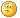#www.vustudents.ning.com

We non-commercial site working hard since 2009 to facilitate learning Read More. We can't keep up without your support. Donate.

Current Mid Term Papers Nov 2011, (Fall 2011)

Current Fall 2011 Papers, November 2011 Papers, Mid Term Fall 2011 Papers, Solved Papers, Solved Past Papers, Solved MCQs

Views: 2320

### Replies to This Discussion

Please Share your Current Papers Questions/Pattern here to help each other. Thanks

Find the point of discontinuing of the given function:   2 Marks

f (x) =    3x + 1

X2-7x+12

Find dx  at (-5,6) if x2 + y=0               2 Marks

dy

Determine whether lim  1 – cos |x| exists or not? 3 Marks

x−>0      x

if f '(t)= 2t then find the intervals on which the function f(t) increasing and decreasing? 3 Marks

Use an epsilon-delta argument to prove that                    5 Marks

lim (3x + 5) = 35

x−>0

if f(x) = x -5x+6 then find the intervals where the function is concave up and concave down.    5 Marks

MTH101_MID TERM LATEST PAPER_2/11/2011

Find the point of discontinuing of the given function: 2 Marks
f (x) = 3x + 1
X2-7x+12

Find dx at (-5,6) if x2 + y2 =0 2 Marks
dy

Determine whether lim 1 – cos |x| exists or not? 3 Marks
x−>0 x

if f '(t)= 2t then find the intervals on which the function f(t) increasing and decreasing? 3 Marks

Use an epsilon-delta argument to prove that 5 Marks
lim (3x + 5) = 35
x−>0

if f(x) = x -5x+6 then find the intervals where the function is concave up and concave down. 5 Marks

budyzz telme mth101 midtrm mcq's past papers sy hn k difrent hn?????????????????